﻿ The Distribution and Uncertainty Quantification of Wind Profile in the Stochastic General Ekman Momentum Approximation Model
 J. Meteor. Res.2019, Vol. 33Issue (2): 336-348PDF
http://dx.doi.org/10.1007/s13351-019-8076-3
The Chinese Meteorological Society
0

#### Article Information

YAN, Bing, Sixun HUANG, Jing FENG, et al., 2019.
The Distribution and Uncertainty Quantification of Wind Profile in the Stochastic General Ekman Momentum Approximation Model. 2019.
J. Meteor. Res., 33(2): 336-348
http://dx.doi.org/10.1007/s13351-019-8076-3

### Article History

in final form December 26, 2018
The Distribution and Uncertainty Quantification of Wind Profile in the Stochastic General Ekman Momentum Approximation Model
Bing YAN1, Sixun HUANG1,2, Jing FENG1, Yu WANG1
1. College of Meteorology and Oceanography, National University of Defense Technology, Nanjing 211101;
2. Center for Computational Science and Finance, Shanghai University of Finance and Economics, Shanghai 200433
ABSTRACT: The general Ekman momentum approximation boundary-layer model (GEM) can be effectively used to describe the physical processes of the boundary layer. However, eddy viscosity, which is an approximated value, can lead to uncertainty in the solutions. In this paper, stochastic eddy viscosity is taken into consideration in the GEM, and generalized polynomial chaos is used to quantify the uncertainty. The goal of uncertainty quantification is to investigate the effects of uncertainty in the eddy viscosity on the model and to subsequently provide reliable distribution of simulation results. The performances of the stochastic eddy viscosity and generalized polynomial chaos method are validated based on three different types of eddy viscosities, and the results are compared based on the Monte Carlo me-thod. The results indicate that the generalized polynomial chaos method can be accurately and efficiently used in uncertainty quantification for the GEM with stochastic eddy viscosity.
Key words: generalized polynomial chaos     Monte Carlo method     general Ekman momentum approximation model     Ekman spiral
1 Introduction

Boundary-layer issues have received considerable and critical attention in the fields of meteorology and oceanography (Mahrt, 1998; Tan, 2001; Baklanov et al., 2011). The boundary layer is a complex system that consists of complicated dynamic and thermodynamic processes (Tan and Wu, 1994). Ekman (1905) derived the Ekman model, although the Ekman model is a simple boundary-layer model, it can capture the main physical characteristics of the atmosphere and ocean. The Ekman model has been widely applied to theoretical studies of the atmosphere and ocean dynamics (Blumen and Wu, 1983; Twigg and Bannon, 1998; Beare and Cullen, 2010). The deficiency of this approach is that it fails to consider nonlinear characteristics, whereas the nonlinear features in the actual atmospheric boundary layer are not negligible. Intermediate models have been developed over time. These models are simpler than complete boundary-layer model but have less dynamic constrains than the Ekman model (Tan and Wang, 2002). Wu and Blumen (1982) revised the Ekman model with the geostrophic momentum approximation, in which the inertial term is taken into consideration. The modified boundary-layer model with the Ekman momentum approximation was proposed by Tan and Wu (1994). Cullen (1989) developed a semi-geostrophic boundary-layer model that is more accurate than the Ekman model for the two-dimensional boundary-layer problem. Tan and Wang (2002) extended the Ekman momentum approximation to the general Ekman momentum approximation (GEM), in which the turbulent friction term is complete. Recently, many new achievements have been made by scholars in the field. Beare and Cullen (2010) incorporated well-mixed boundary layers into a semi-geostrophic model, which broadened the applications of the model. Marlatt et al. (2012) evaluated two closure models with a direct numerical simulation at a Reynolds number of 1000. Of these two models, the O’Brien model that requires data both at the surface layer and at the top of the boundary layer proved superior.

For the case of eddy viscosity, Berger and Grisogono (1998) provided an approximate solution for the baroclinic Ekman boundary layer with variable eddy viscosity using the WKB (Wentzel–Kramers–Brillouin) method. Tan and Wang (2002) analysed the wind structure of the baroclinic and variable eddy diffusivity semi-geostrophic Ekman boundary layer. The approximate analytical solution for the weakly nonlinear Prandtl model for simple slope flows with variable eddy diffusivities was derived by Grisogono et al. (2015). The retrieval of the eddy thermal conductivity in the weakly nonlinear Prandtl model for katabatic flows was recently discussed by Yan et al. (2017).

Because errors are unavoidable, including the model error and the observation error, we consider the eddy viscosity as an uncertain quantity, which satisfies some distribution and subsequently obtains the distribution of wind profiles by uncertainty quantification. Berner et al. (2017) reviewed the development of stochastic parameterized scheme and proposed that the introduction of the stochastic parameterization schemes better shows the uncertainty of the model, which can improve the quantification of forecast uncertainty. The methods of uncertainty quantification have been extensively developed and are still being widely researched. Such methods include the Monte Carlo method, perturbation methods, moment equations, and generalized polynomial chaos (gPC; Xiu, 2010).

Monte Carlo sampling is one of the most widely used uncertainty methods. The realizations of the random variables are sampled based on the given probability distribution function. For each sample, the data are fixed, and the model is deterministic. Statistical information, e.g., the mean and variance, can be extracted from the ensemble of the deterministic model solutions. The advantages of the Monte Carlo method are that it is simple and straightforward, and there is no need to modify the deterministic solvers. However, a large number of samples and computationally intensive deterministic codes are required to obtain accurate statistical information. Recently, Monte Carlo techniques have been developed to accelerate convergence, and such techniques include Latin hypercube sampling (Loh, 1996; Helton and Davis, 2003), quasi-Monte Carlo sampling (Fox, 1999; Xiu, 2009), and so on. However, their applicability is limited because of the inherent limitations of these methods. Perturbation methods are prevalent non-sampling methods (D’Onofrio et al., 2017). In perturbation methods, the random variables are expanded via a Taylor series, and the series is truncated at a certain order, which is usually the second order, because the system becomes extremely complicated beyond the second order. This method has been applied in various engineering fields. A natural restriction of this method is that the magnitude of the uncertainties cannot be overly large (typically less than 10%). In the moment equation approach, the moments of the random output are directly computed (Singer, 2006; Barzel and Biham, 2011). The closure problem of the equations is the largest limitation, and this issue often requires information about higher moments. A recently developed method, the generalized polynomial chaos expansion, has become one of the most commonly used methods (Li and Xiu, 2009; Zeng and Zhang, 2010; Li et al., 2014; Wang et al., 2018; Yan et al., 2018). The random output can be expanded as orthogonal polynomials of the random input parameters using gPC. Essentially, this process is a spectral expansion in random space. When the solution smoothly depends on the random input parameters, the convergence rate of this method will be fast.

To solve for the undetermined gPC coefficients of the solutions, two types of methods are commonly adopted: intrusive (e.g., stochastic Galerkin method) (Marzouk and Najm, 2009; Najm, 2009) and non-intrusive methods (e.g., regression method and stochastic collocation method) (Li et al., 2009; Lin et al., 2010). The advantage of the intrusive approach is that it can directly solve the coefficients. The main limitation of the intrusive approach, however, is the modification of the existing deterministic code. Generally, the code used to solve the deterministic models is complex, which makes the implementation of the intrusive method difficult. Unlike the intrusive method, the deterministic code does not need to be revised during the implementation of the non-intrusive method. In this study, the non-intrusive method is used to ascertain the undetermined gPC coefficients of the solutions.

In the present study, we analyze the randomness of eddy viscosity and quantify the uncertainty of wind profiles by the GEM. Generalized polynomial chaos is selected for the uncertainty quantification of the stochastic boundary-layer model, and the regression method is used to determine the gPC coefficients. The overall structure of the study is covered in the following five sections: Section 1 is an introductory chapter; Section 2 presents the GEM; Section 3 focuses on generalized polynomial chaos and the implementation of the regression method; Section 4 presents the results of the numerical experiment; and Section 5 provides a summary and discussion.

2 The GEM

The classic Ekman model has been widely used in theoretical research of the atmosphere and ocean dynamics (Blumen and Wu, 1983; Twigg and Bannon, 1998; Beare and Cullen, 2010). Tan and Wang (2002) improved the classic Ekman model by including the effect of the inertia terms and variable eddy viscosity. This modification maintains the computational simplicity and the inherent nonlinearity of the system. The GEM is given as follows:

 $\left\{ \begin{array}{l} \dfrac{\partial }{{\partial z}}(K\dfrac{{\partial u}}{{\partial z}}) + {a_1}u + {b_1}v = {c_1}, \\ \dfrac{\partial }{{\partial z}}(K\dfrac{{\partial v}}{{\partial z}}) + {a_2}u + {b_2}v = {c_2}, \\ \end{array} \right.$ (1)

where $K$ is the vertical eddy viscosity coefficient and $(u, v)$ represent the x- and y-components of wind speed, respectively.

 $\left\{ \begin{array}{l} {a_1} = - \lambda \dfrac{{\partial {u_{\rm{e}}}}}{{\partial x}}, {b_1} = f - \lambda \dfrac{{\partial {u_{\rm{e}}}}}{{\partial y}}, {c_1} = f{v_{\rm{g}}} + \lambda \dfrac{{\partial {u_{\rm{e}}}}}{{\partial t}}, \\ {a_2} = - (f + \lambda \dfrac{{\partial {v_{\rm{e}}}}}{{\partial x}}), {b_2} = - \lambda \dfrac{{\partial {v_{\rm{e}}}}}{{\partial y}}, {c_2} = - f{u_{\rm{g}}} + \lambda \dfrac{{\partial {v_{\rm{e}}}}}{{\partial t}}. \\ \end{array} \right.$ (2)

In Eq. (2), $\lambda$ is a control parameter. If $\lambda$ = 0, Eq. (1) reduces to the classic Ekman model, but when $\lambda$ = 1, Eq. (1) becomes the modified Ekman boundary-layer model with the general Ekman momentum approximation. In this paper, we set $\lambda$ = 1; $f$ is the Coriolis parameter, which is assumed to be constant in this study; $({u_{\rm{e}}}, {v_{\rm{e}}})$ are the solution to the classic Ekman model (Tan et al., 2006), and $({u_{\rm{g}}}, {v_{\rm{g}}})$ are the geostrophic wind. The boundary conditions of Eq. (1) are given by ( ${\left. u \right|_{z = 0}} = 0, {\left. v \right|_{z = 0}} = 0$ ) and ( ${\left. u \right|_{z = {H_{\rm{b}}}}} = {u_{\rm{T}}}, {\left. v \right|_{z = {H_{\rm{b}}}}} = {v_{\rm{T}}}$ ), where ${H_{\rm{b}}}$ is the depth of the atmospheric boundary layer, and $({u_{\rm{T}}}, {v_{\rm{T}}})$ are the wind speed at the top of the boundary layer (Tan et al., 2006), which can be obtained by the geostrophic momentum approximation model. We discretize the GEM in space and time and then numerically solve the model.

This study focuses on the randomness of the stochastic eddy viscosity, and we change the form of $K$ from $K(z)$ to $K(z, { \xi})$ in Eq. (1). The eddy viscosity is not only a function of $z$ but also a function of the random variable ${ \xi}$ .

3 The gPC and implementation of the regression method 3.1 Generalized polynomial chaos

The original concept of polynomial chaos in uncertainty quantification was proposed by Wiener (1938). Inspired by this idea, Ghanem and Spanos (1991) expanded polynomial chaos to generalized polynomial chaos and applied it to stochastic linear equations for the first time. This method has become one of the most widely used techniques in uncertainty analysis. A brief description of generalized polynomial chaos is in the Appendix.

We can expand $(u, v)$ with the gPC approach:

 $\begin{array}{l} u( \xi) = \displaystyle\sum\limits_{i = 0}^P {{u_i}{\varPsi _i}( \xi)}, \\ v( \xi) = \displaystyle\sum\limits_{i = 0}^P {{v_i}{\varPsi _i}( \xi)}, \\ \end{array}$

where $P$ is the number of total orders, ${\varPsi _i}( { \xi})$ is the multi-dimensional polynomial chaos and ${ \xi}$ is random vector, which consists of mutually independent random variables. Solving the stochastic GEM is transformed into solving for the expansion coefficients $({u_i}, {v_i})$ . The statistical information associated with the output variables $(u, v)$ is based on the coefficients; for example, the statistical information for the wind speed $u$ is as follows: the expectation ${\rm E}\left[ u \right] = {u_0}$ and variance ${\rm Var}\left[ u \right] = \displaystyle\sum\limits_{i = 1}^P {{\rm E}\left[ {\varPsi _i^2} \right]{{({u_i})}^2}}$ . The form is similar for wind speed $v$ .

3.2 Regression method

The coefficients of the output variables can be determined by various methods. Numerical methods include intrusive methods, such as the stochastic Galerkin method (Marzouk and Najm, 2009; Najm, 2009), and non-intrusive methods. We substitute the input parameters and output variables with the form of gPC expansion. Then, using the feature of the inner product of the orthogonal polynomials, we apply a Galerkin projection to each basis polynomial. Because the basis polynomials are fixed according to the probability distribution of the input parameters, the key problem is the determination of coefficients. After the coefficients are determined, the approximate statistics of the solution can be computed. One major drawback of the stochastic Galerkin method is that the implementation is cumbersome. Additionally, the formula needs to be derived; hence, the associated codes must be rewritten for the larger, coupled system. When the origin model is complex and highly nonlinear, the explicit derivation of the stochastic Galerkin approach can be nontrivial and sometimes impossible. Thus, another numerical scheme is adopted in this study, which is the non-intrusive method (Li et al., 2009; Lin et al., 2010). A common non-intrusive method is the regression method. In this approach, the algorithms are straightforward and easy to implement. In addition, the model is not affected by the complexity or nonlinearity of the original model as long as the deterministic code is reliable. The regression procedures for the stochastic GEM are given as follows.

The stochastic GEM is given in Eq. (3):

 $\left\{ \begin{array}{l} \dfrac{\partial }{{\partial z}}(K(z, \xi)\dfrac{{\partial u}}{{\partial z}}) + {a_1}u + {b_1}v = {c_1}, \\ \dfrac{\partial }{{\partial z}}(K(z, \xi)\dfrac{{\partial v}}{{\partial z}}) + {a_2}u + {b_2}v = {c_2}, \\ \end{array} \right.$ (3)

where $K(z, \xi)$ is the height-dependent random eddy viscosity and the other symbols in Eq. (3) are the same as those in Eq. (1). The basic concept of this approach is as follows.

Step 1: generate the realizations ${ \xi ^j}(j = 1, ..., N)$ based on their prescribed probability distribution.

Step 2: calculate each realization of input parameter ${K^j}(j = 1, 2, ..., N)$ according to the gPC expansion of $K$ and run the deterministic code for each input parameter. The following realization solution is obtained: $({u^j}, {v^j}), j = 1, 2, ..., N$ . Next, build the matrix of gPC polynomials.

Step 3: establish the overdetermined equations based on the results of Step 2.

 $\left({\begin{array}{*{20}{c}} {{\varPsi _0}({{ \xi }^1})}& \ldots &{{\varPsi _P}({{ \xi }^1})} \\ \vdots & \ddots & \vdots \\ {{\varPsi _0}({{ \xi }^N})}& \cdots &{{\varPsi _P}({{ \xi }^N})} \end{array}} \right)\left(\begin{array}{l} {u_0} \\ \vdots \\ {u_P} \\ \end{array} \right) = \left(\begin{array}{l} u({{ \xi }^1}) \\ \vdots \\ u({{ \xi }^N}) \\ \end{array} \right).$

The number of collocation points/realizations should be at least twice of the number terms retained in the expansion [i.e., $N > 2(P + 1)$ ] (Sun and Sun, 2015).

Step 4: obtain the gPC expansion coefficients by solving the overdetermined equations in Step 3. The least squares method is used to solve the equations.

It is worth noting that its corresponding coefficients $({u_i}, {v_i})$ are functions of $(x, y, z, t)$ , so two overdetermined equations must be solved at spatial location and point in time.

A flow chart of the calculation procedure is provided in Fig. 1.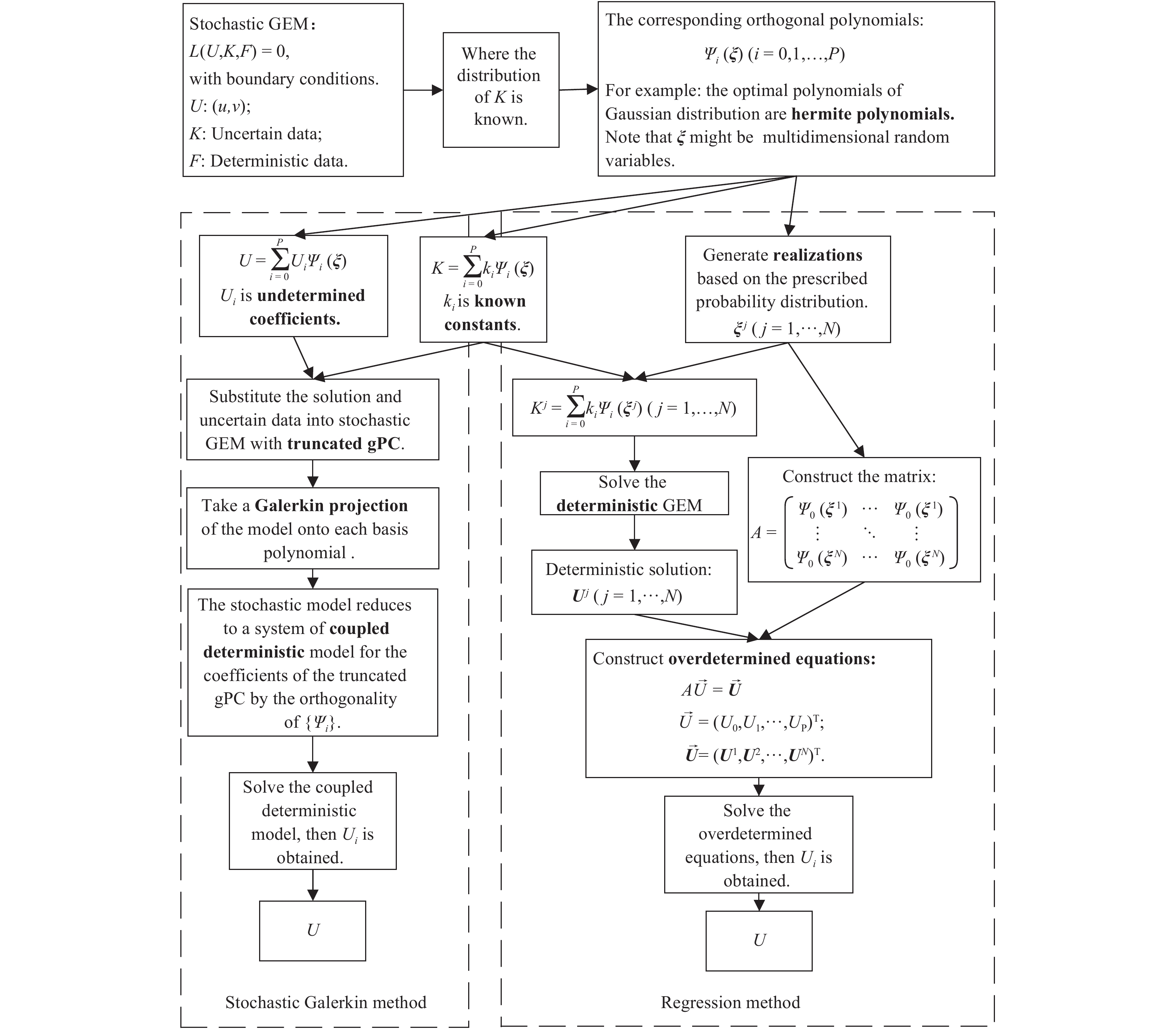Figure 1 The flow chart for solving the GEM with stochastic eddy viscosity based on gPC. The left chart is the stochastic Galerkin method; the right chart is the stochastic collocation method. The number of samples should be at least twice the number of expansion terms [ $N > 2(P + 1)$ ].
4 Numerical experiment

To examine the reliability and computational efficiency and make the procedure convenient to implement, a typical surface geostrophic shear flow and three types of random eddy viscosities are chosen for analysis. Each type is assessed based on the Monte Carlo method and the deterministic model.

The geostrophic shear flow is given as follows (Tan and Wang, 2002):

 ${u_{\rm{g}}} = {u_0} - \alpha y, \, \, {v_{\rm{g}}} = 0,$

where ${u_0}$ = 20 m s–1 and $\alpha$ is a constant. We set $\alpha = \pm 0.4f$ and $f = {10^{ - 4}}\;{{\rm{s}}^{{\rm{ - 1}}}}$ : $\alpha = 0.4f > 0$ denotes the cyclonic shear flow, and $\alpha = - 0.4f < 0$ is the anticyclonic shear flow. In this paper, ${H_{\rm{b}}}$ in Eq. (1) is assumed to be 1500 m (Tan and Wang, 2002).

Three different types of random eddy viscosities are considered. In Type I, eddy viscosity is assumed to be a function that varies with height, and some parameters in the function are uncertain. In Type II, eddy viscosity is a vertically varying random parameter, and the mean is constant and height independent. In Type III, eddy viscosity is a vertically varying random parameter, and the mean is a function that varies with height. The Gaussian distribution is selected in this paper. The corresponding optimal orthogonal polynomials are Hermite polynomials. The order of truncated gPC expansion is $p = {\rm{4}}$ in this study. Combining with the dimension number of random variables, we can calculate the number of orthogonal polynomials ${P}$ , and according to $N > 2 ({P} + 1)$ , we set $N = 2.5 \times ({P} + 1)$ . The number of executions in the Monte Carlo method is 5000.

4.1 Experiment Ⅰ

We assume $K(z, \omega) = {K_0}(1 + \delta z){\rm{e}^{{{ - \delta z} / {(1 + \delta {z_{\rm{m}}})}}}}$ (Tan, 2001), where ${K_0} = 0.3 \, {{\rm{m}}^2} {{\rm{s}}^{ - 1}}$ , $\delta \sim {\cal{N}}(0.2, {0.05^2})$ m–1, and ${z_{\rm{m}}} \sim$ ${\rm{{\cal N}}}(500,{50^2})$ m, where ${\cal{N}}$ is for Gaussian distribution. The probability distribution function is as follows.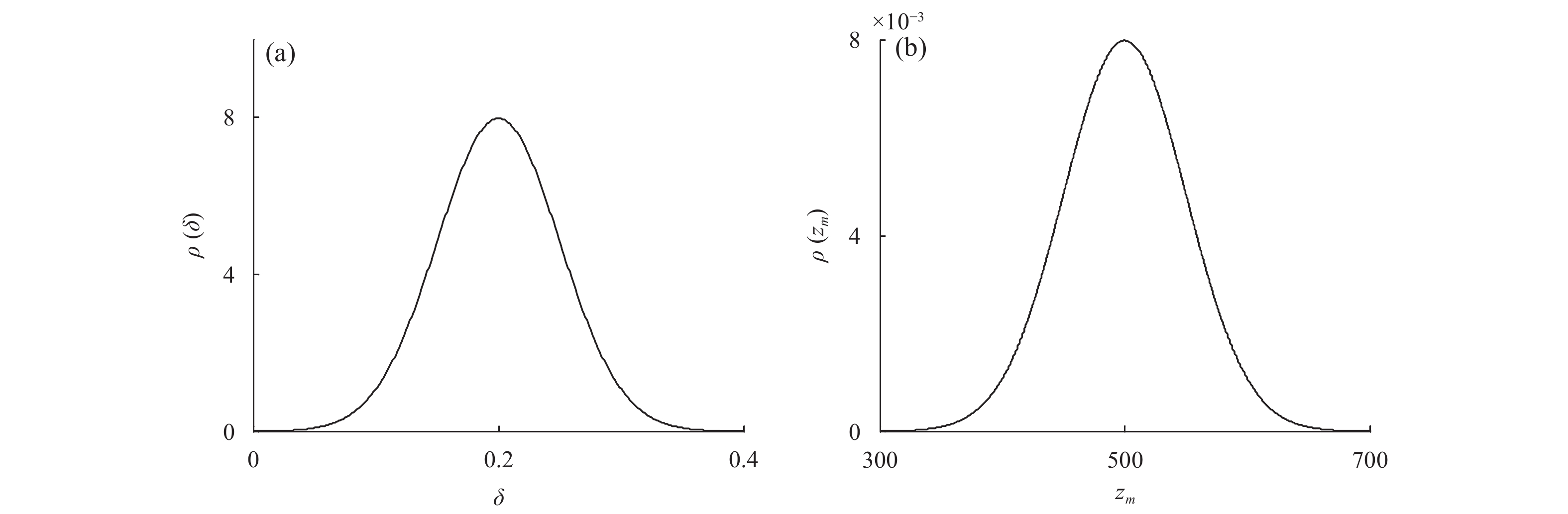Figure 2 Probability density functions of (a) δ and (b) ${z_{\rm{m}}}$ . The approximate range of δ is (0, 0.4), and that of ${z_{\rm{m}}}$ is (300, 700).

The orthogonal Hermite polynomials of the two-dimensional Gaussian random variables truncated at the third order are given as follows (Sun and Sun, 2015) (more details about derivation process is in the Appendix):

 \begin{align} & {\varPsi _{\rm{0}}}{\rm{ = 1;}}\\ & {\varPsi _{\rm{1}}}{\rm{ = }}{\xi _{\rm{1}}},{\varPsi _{\rm{2}}}{\rm{ = }}{\xi _{\rm{2}}};\\ & {\varPsi _{\rm{3}}}{\rm{ = }}\xi _{\rm{1}}^{\rm{2}}{\rm{ - 1}},{\varPsi _{\rm{4}}}{\rm{ = }}{\xi _{\rm{1}}}{\xi _{\rm{2}}},{\varPsi _{\rm{5}}}{\rm{ = }}\xi _{\rm{2}}^{\rm{2}}{\rm{ - 1}},{\varPsi _6} \!= \xi _{\rm{1}}^3{\rm{ - 3}}{\xi _1;} \\ & {\varPsi _7} \!= {\xi _2}({\xi _1}^2 - 1),{\varPsi _8} \!=\! {\xi _1}({\xi _2}^2 - 1), \!{\varPsi _9} \!=\! \xi _2^3{\rm{ - 3}}{\xi _2}, \end{align}

where $({\xi _1}, {\xi _2})$ are two-dimensional independent random variables based on the standard normal distribution. According to the transformation formula of standard normal distribution and normal distribution, the gPC expansions of $\delta$ and ${z_{\rm{m}}}$ are as follows:

 $\begin{array}{l} \delta = 0.2{\varPsi _{\rm{0}}} + 0.05{\varPsi _{\rm{1}}}, \\ {z_{\rm{m}}} = 500{\varPsi _{\rm{0}}} + 50{\varPsi _{\rm{2}}}. \\ \end{array}$

We conduct the experiment according to the procedure shown in Fig. 1. The result is shown in Fig. 3.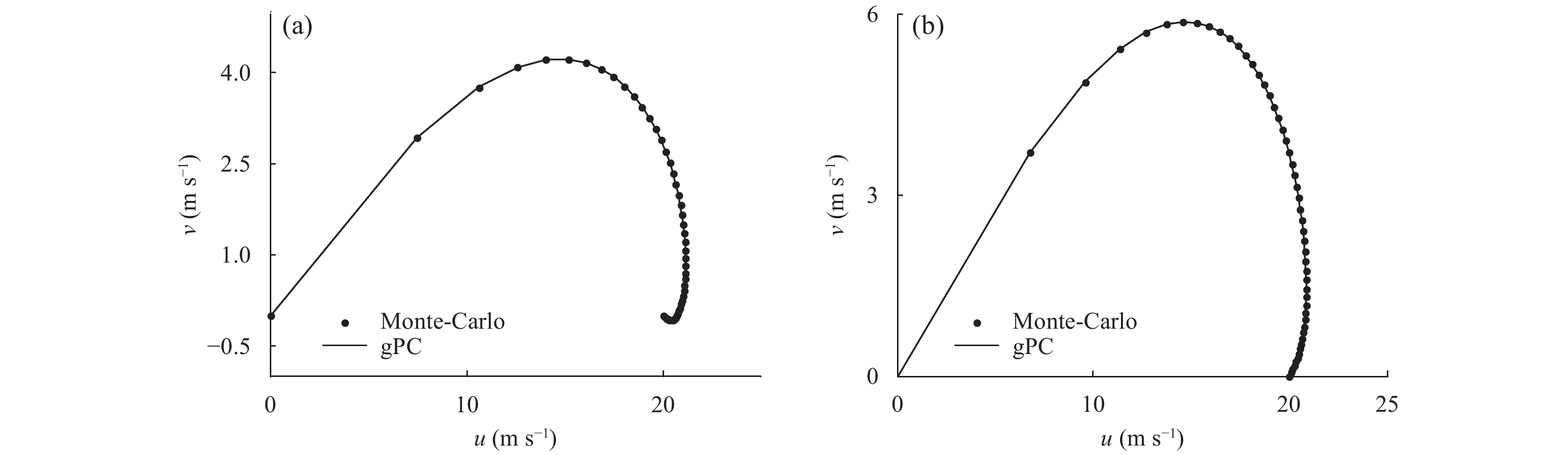Figure 3 Average Ekman spiral comparison for (a) cyclonic and (b) anticyclonic shear flows in experiment I. The solid and dotted lines represent the results obtained by using the gPC and Monte Carlo methods, respectively.

The average of wind speed distributions calculated by gPC and Monte Carlo methods are consistent with the rule of Ekman spiral. The average Ekman spirals of two methods are visually indistinguishable.

The central processing unit (CPU) running time (s) and root-mean-square error (RMSE; m s–1) are given in Table 1.

Table 1 The CPU time and RMSE of the two methods
 Method Cyclonic Anticyclonic CPU time RMSE CPU time RMSE gPC 0.12 0.069 0.12 0.079 Monte Carlo 5.85 0.078 5.90 0.085

Table 1 displays the RMSE of the two methods, and the two circulation types are approximately equal. The order of the RMSE is 10–2, and the errors of cyclonic type are slightly smaller than the anticyclonic ones. The CPU time for the gPC is much less than that required by the Monte Carlo method (approximately 1/50 the time of the latter). These results validate the reliability and speed of the gPC method in solving the GEM with stochastic eddy viscosity.

Figures 4 and 5 illustrate that the standard deviation of the wind speed is less than 1. There are some differences between the two methods, but these differences are small. This result indicates that gPC is an efficient method for solving the GEM with a random eddy viscosity function. The maximum value of standard deviation of $u$ appears about 300 m above the ground. The curve in Fig. 4b is smoother than Fig. 4a, which means the variation of standard deviation of $u$ in cyclonic shear flow is greater than that in anticyclonic shear flow. The maximum standard deviation of $v$ exist in the middle-level of boundary layer, the standard deviation of anticyclonic shear flow type is greater than that of cyclonic shear flow.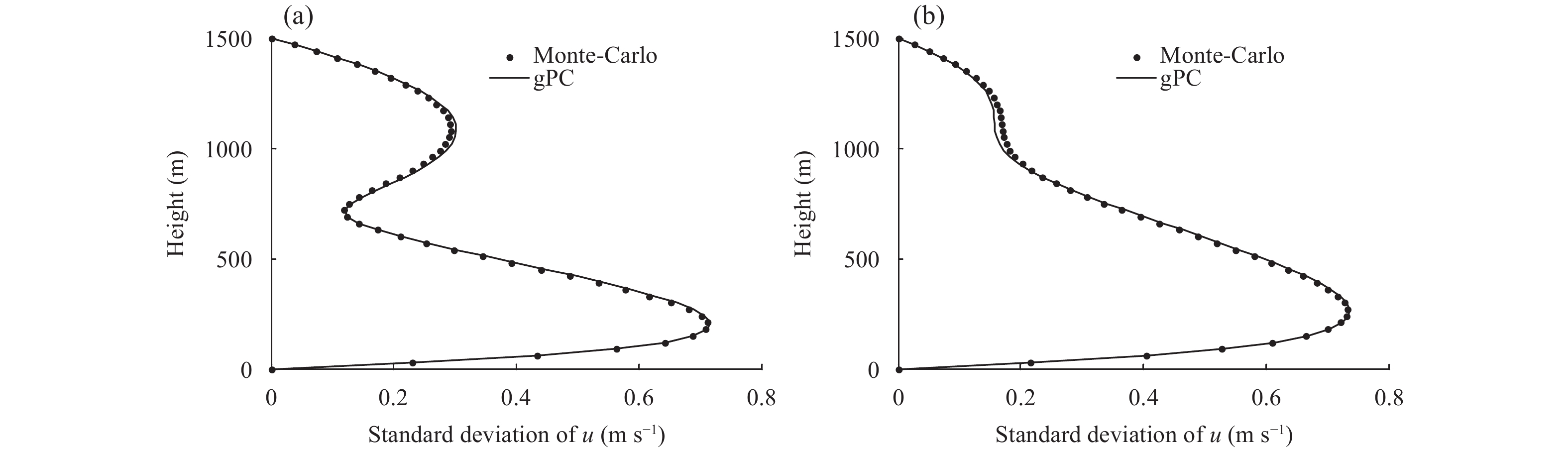Figure 4 As in Fig. 3, but for the standard deviation of u.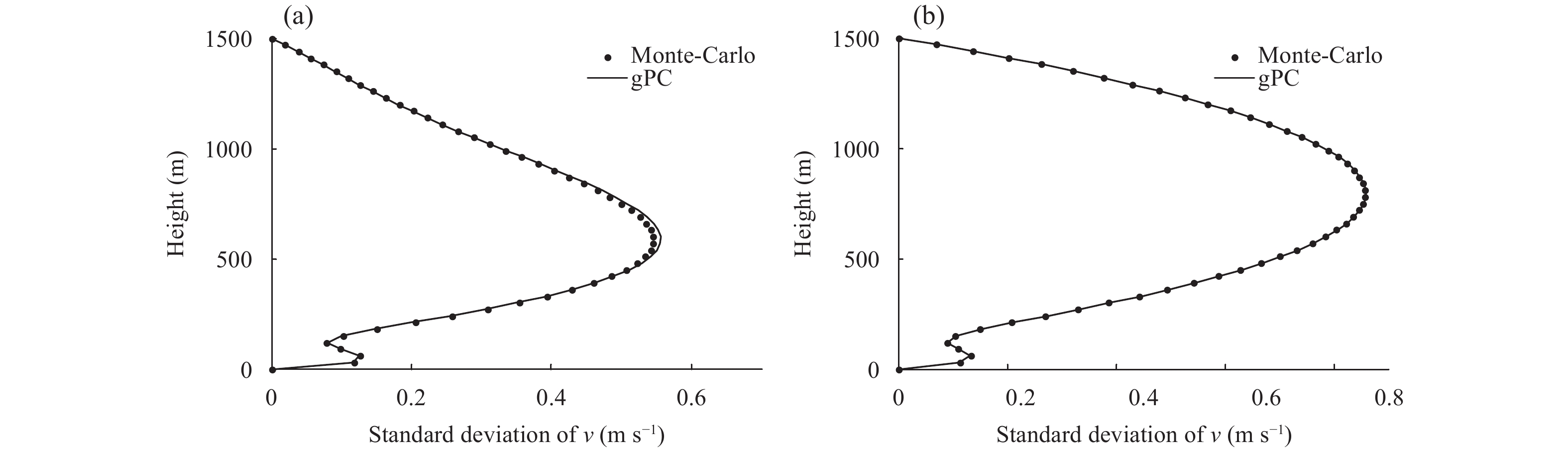Figure 5 As in Fig. 3, but for the standard deviation of v.

Figures 6 and 7 illustrate that large uncertainty of wind speed appear in the middle of the boundary layer. The uncertainty of zonal wind is less than that of meridional wind; the uncertainty of wind speed in cyclonic shear flow is less than that in anticyclonic shear flow.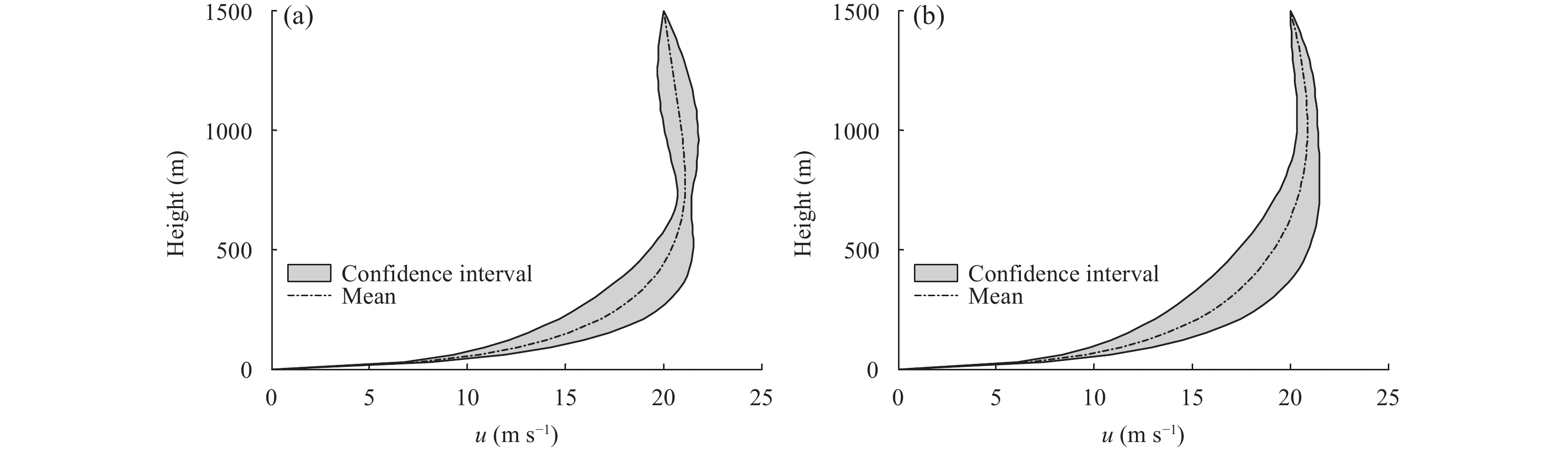Figure 6 The 99% confidence interval of u for (a) cyclonic and (b) anticyclonic shear flow in experiment I. The grey shaded area is the confidence interval of wind speed, and the dotted line is the mean of wind distribution.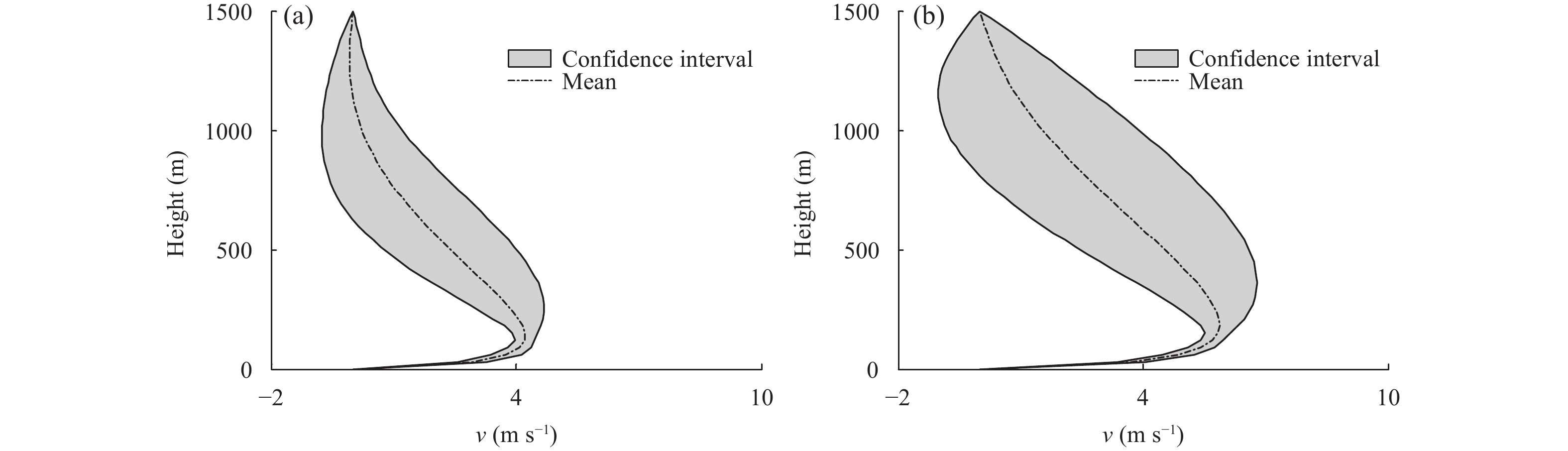Figure 7 As in Fig. 6, but for v.
4.2 Experiment Ⅱ

We set the eddy viscosity to a vertically varying random parameter with a constant mean of $\bar K(z) = {\rm const}$ . The dimension of the random variables is the same as the number of vertical points. Because the number of vertical points is enormous, the random variables become high-dimensional, and the number of terms retained in the expansion sharply increases. Therefore, we must reduce the dimension of the random variables. Assuming that the random field $K$ is characterized by its mean and covariance function, we obtain the following formulas:

 $\bar K(z) = 8, \quad {C_K}({z_1}, {z_2}) = {{\rm e}^{ - {{\left| {{z_1} - {z_2}} \right|} / c}}}, \quad {z_1}, {z_2} \in [ - a, a],$ (4)

where $c > 0$ is the correlation length. We choose $\bar K(z) = 8$ to ensure that the random fluctuations do not result in $K \leqslant 0$ over any part of the domain. Additionally, we assume $a = 25$ and $c = 0.01$ .

We represent $K$ using a truncated Karhunen–Loève (K–L) expansion (Xiu, 2010).

 $K(z, \omega) = \bar K(z) + \sum\limits_{j = 1}^N {\sqrt {{\lambda _j}} {\phi _j}(z){\xi _j}}.$

The eigenpairs $\left\{ {{\lambda _j}, {\phi _j}} \right\}$ can be obtained by solving the following integral equation.

 $\int\limits_{ - a}^a {{{\rm e}^{ - {{\left| {{z_1} - {z_2}} \right|} / c}}}{\phi _j}({z_2}){\rm d}{z_2}} = {\lambda _j}{\phi _j}({z_1}).$ (5)

The detailed procedure for solving Eq. (11) can be seen in the associated literatures (Le Maître and Knio, 2010; Xiu, 2010). Through this process, we can decrease the high dimensionality of the random variables.

In this study, we use three-dimensional random variables to represent $K(z, \xi)$ and ${\xi _j} \sim {\cal{N}}(0, {2^2}), \;j = 1, 2, 3$ . Note that ${\xi _j}$ is mutually independent. The histogram of $K$ is plotted by generating 10,000 samples of ${\xi _j}$ . The approximate range of $K$ is in Fig. 8, basically conforms to our understanding of this parameter.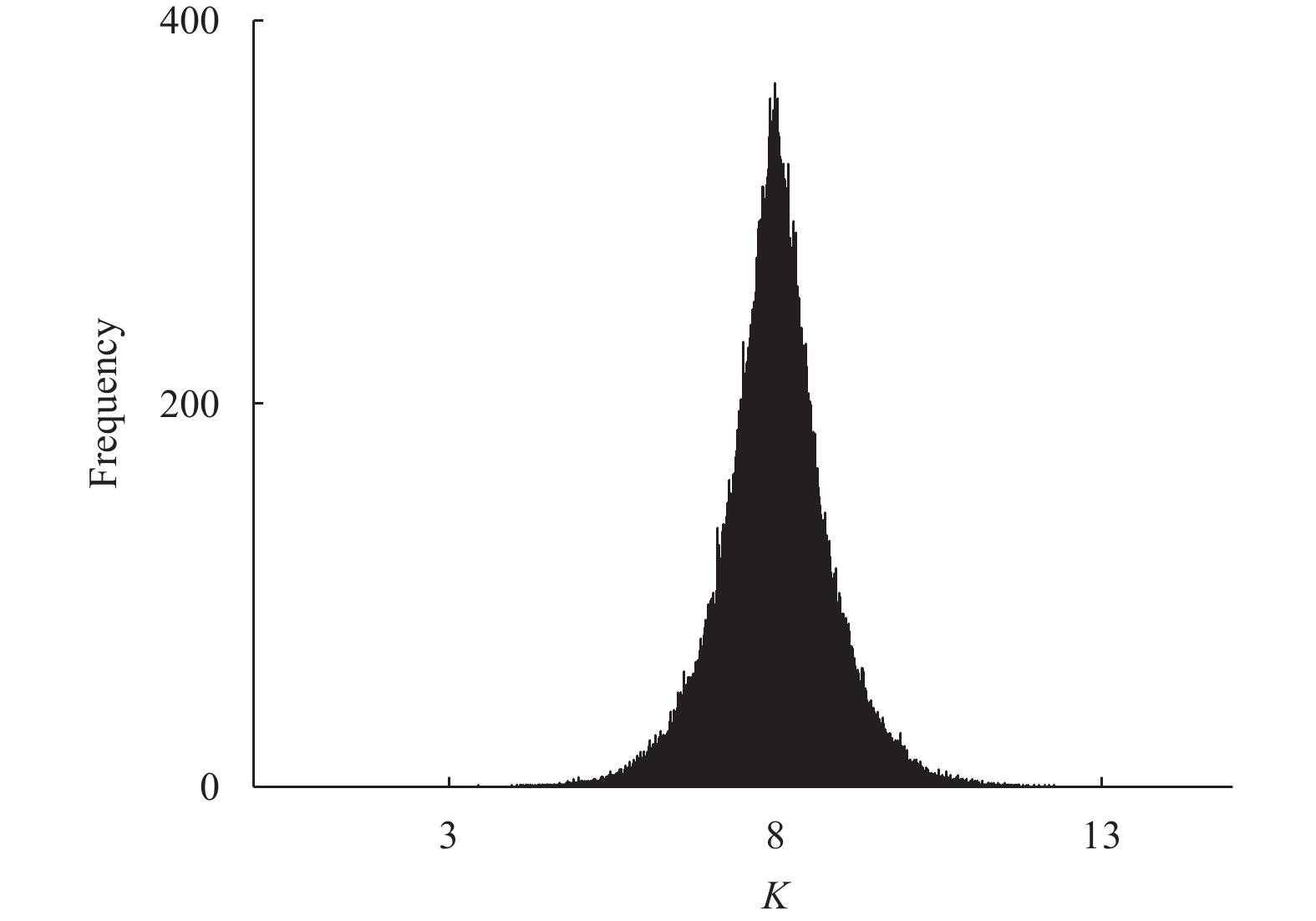Figure 8 The histogram of K. The abscissa is the eddy viscosity, and the ordinate is the frequency. The approximate range of K is (3, 13), and the mean is 8.

In Fig. 9, the average value of wind speed distribution is conform to Ekman spiral, and the results produced by the gPC and Monte Carlo methods are very consistent.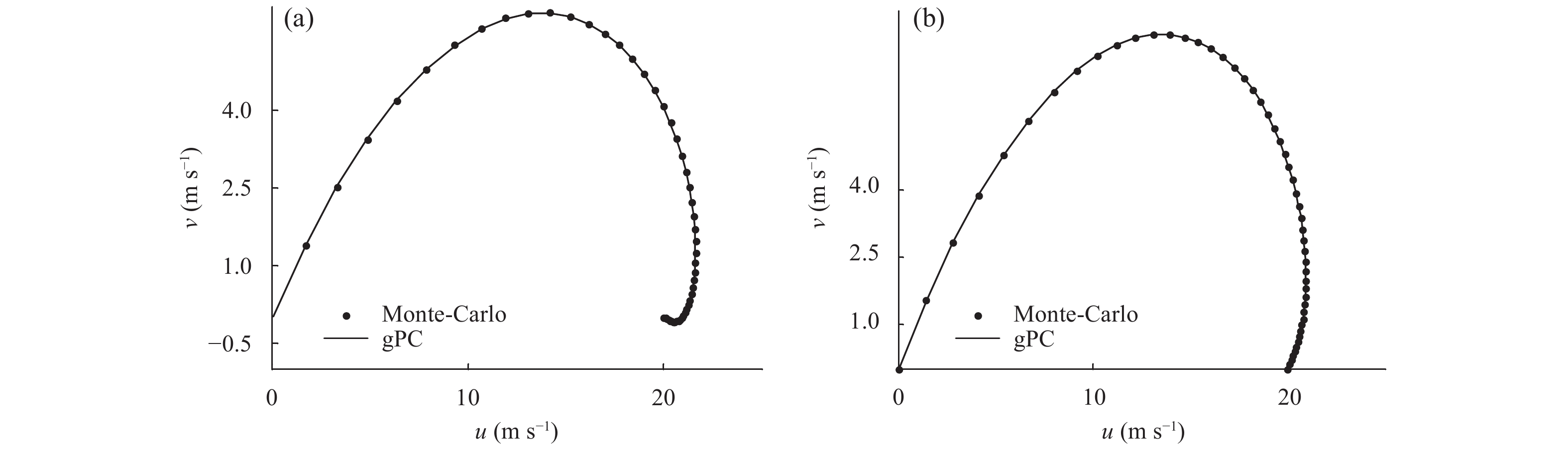Figure 9 As in Fig. 3, but for experiment Ⅱ.

The CPU running time and RMSE can be seen in Table 2. As presented in Table 2, the error order of the two methods is 10–1, which is slightly larger than that from experiment Ⅰ. However, the complexity of experiment Ⅱ is greater than that of experiment Ⅰ because of the higher dimension of the random variables and the truncation error produced by the truncated K-L expansion. The CPU running time for experiment Ⅱ slightly increases compared to that of experiment I, and the computational efficiency of the gPC method is better than that of the Monte Carlo method.

Table 2 The CPU time and RMSE of the two methods
 Method Cyclonic Anticyclonic CPU time RMSE CPU time RMSE gPC 0.18 0.117 0.19 0.143 Monte Carlo 6.06 0.126 5.94 0.148

Similar to experiment Ⅰ, Figs. 10 and 11 illustrate that the standard deviations are less than one. The standard deviation in experiment Ⅱ is less smooth than that in experiment I, which is largely because of the complexity of experiment Ⅱ noted in the analysis of Table 2. Generally, the standard deviations solved by the two methods are very similar, and this result validates the effectiveness of the gPC method. The large values of standard deviations mainly lie at the bottom of the boundary layer. For zonal wind, the standard deviation varies sharply in the lower layer, and for meridional wind, the standard deviation varies sharply in the middle layer of the boundary layer. The standard deviation of cyclones is greater than that of anticyclones. The greater the standard deviation, the higher of error in the deterministic mode.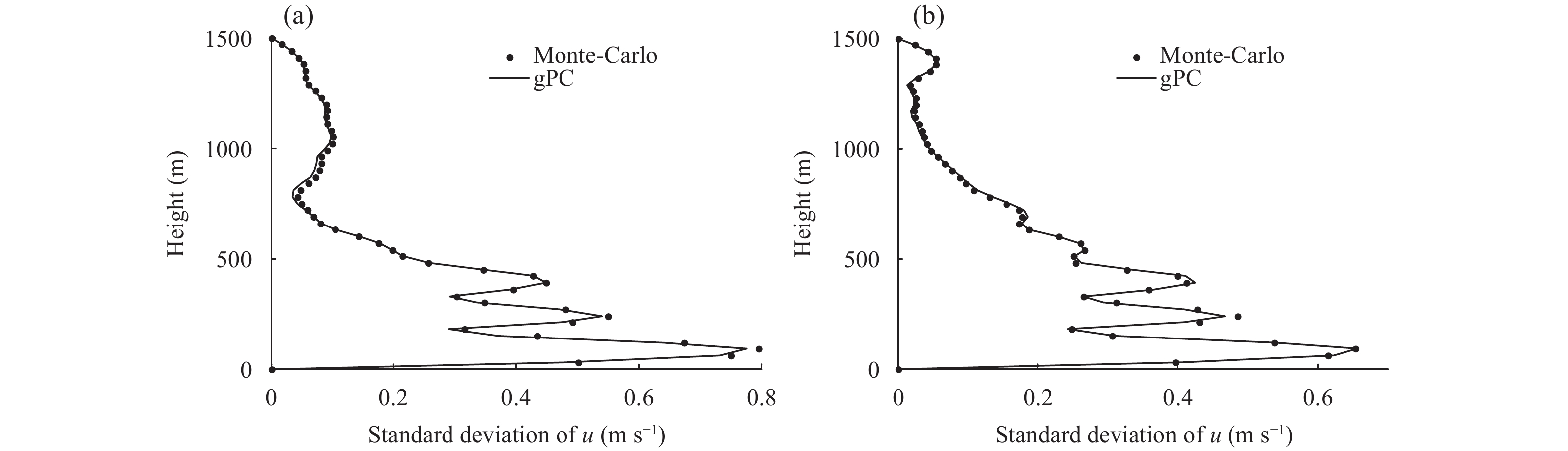Figure 10 As in Fig. 5, but for experiment Ⅱ.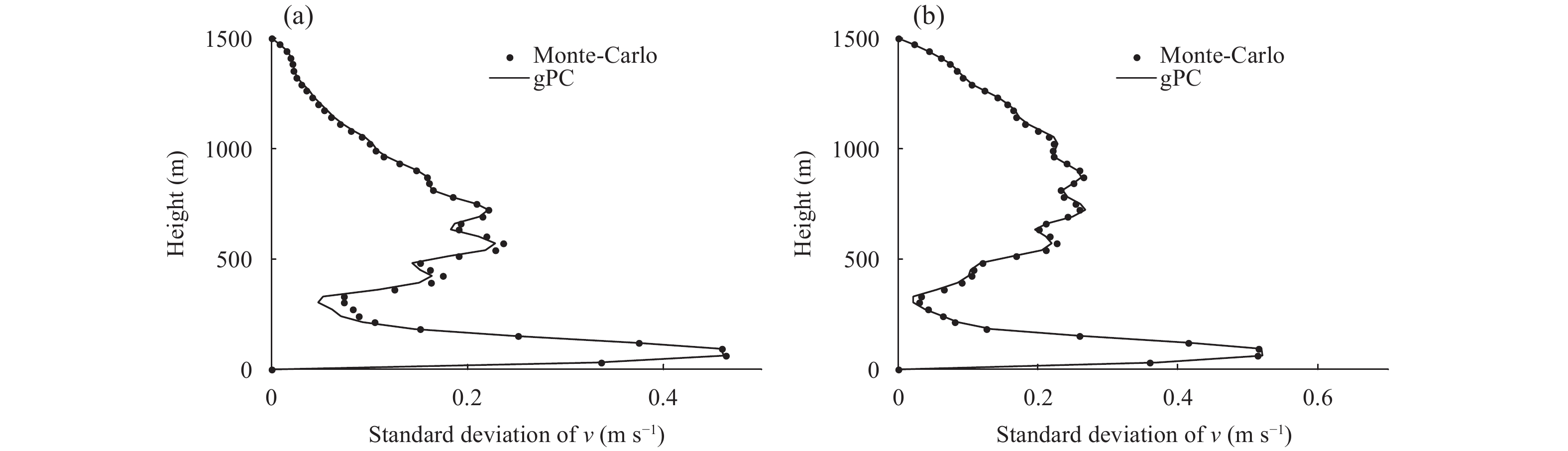Figure 11 As in Fig. 5, but for experiment Ⅱ.

As can be seen from Figs. 12 and 13, the uncertainty of wind speed distribution is small and changes little with height.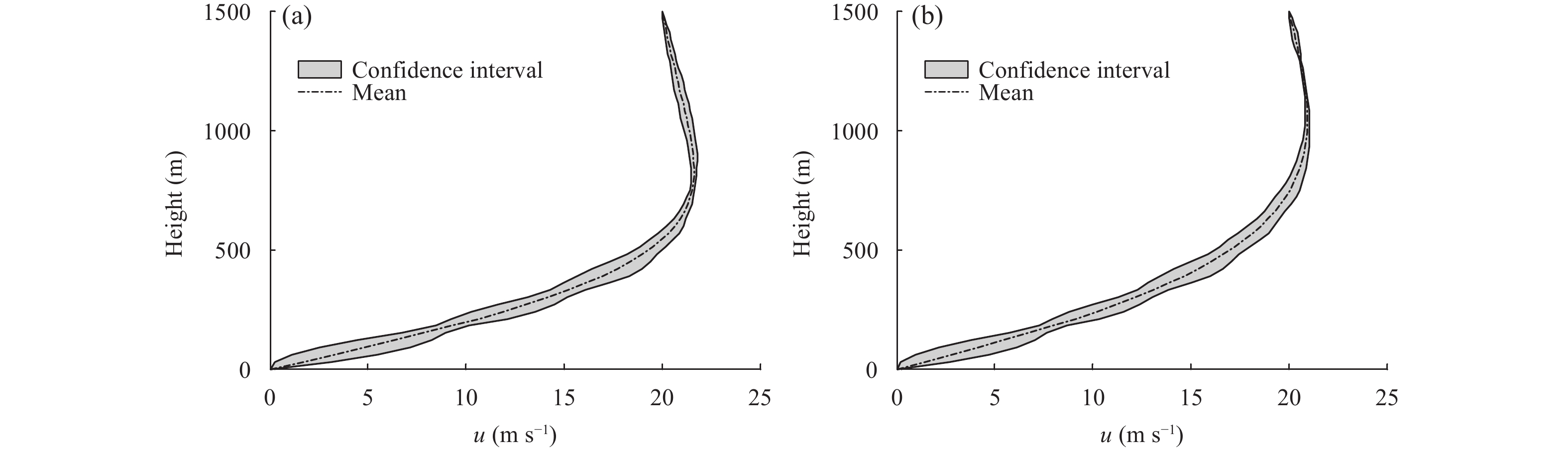Figure 12 As in Fig. 6, but for experiment Ⅱ.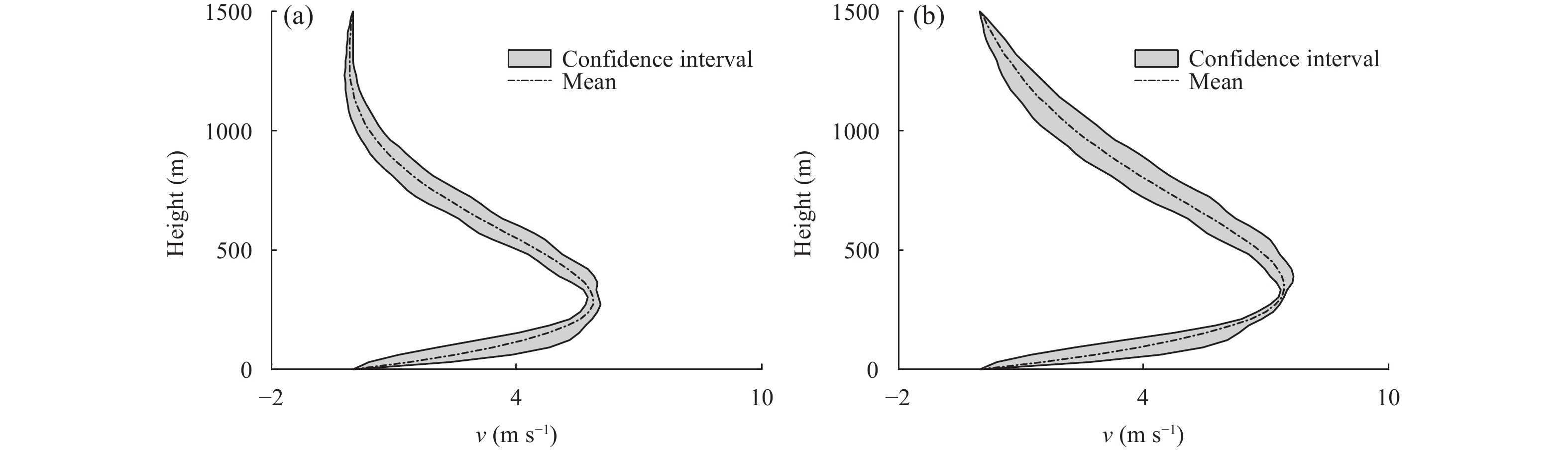Figure 13 As in Fig. 7, but for experiment Ⅱ.
4.3 Experiment Ⅲ

Consider $K(z, \omega) = \bar K(z) + \bar K(z) \times \alpha (z, \xi)/100$ , where $\bar K(z) = {K_0}(1 + \delta z){{\rm e}^{{{ - \delta z} / {(1 + \delta {z_{\rm{m}}}}})}}$ , ${K_0} = 0.3 \, {{\rm{m}}^2} {{\rm{s}}^{ - 1}}$ , $\delta = 0.2\;{{\rm{m}}^{ - 1}}$ , and ${z_{\rm{m}}} = 500\;{\rm{m}}$ . The mean and covariance functions are as follows.

 $\bar \alpha (z) = 0, \quad {C_\alpha }({z_1}, {z_2}) = {{\rm e}^{ - {{\left| {{z_1} - {z_2}} \right|} / c}}}, \quad {z_1}, {z_2} \in [ - a, a],$ (6)

The other parameters in Eq. (12) are the same as those in Eq. (9).

We represent $\alpha$ with a truncated K-L expansion, $\alpha = \bar \alpha +$ $\displaystyle\sum\limits_{j = 1}^N {\sqrt {{\lambda _j}} {\phi _j}{\xi _j}}$ , and truncate the expansion at three-dimensional random variables. Note that ${\xi _j}(j = 1, 2, 3)$ and ${\xi _j} \sim {\cal{N}}(0, {10^2})$ are mutually independent. The histogram of $\alpha$ is then plotted by generating 10,000 samples of ${\xi _j}$ .

The level of random eddy viscosity deviation from the average can be seen in Fig. 14. The results are shown below. In Fig. 15, the average value of wind speed distribution is coincidence with Ekman spiral, and the results of the gPC method are very similar to those of the Monte Carlo method.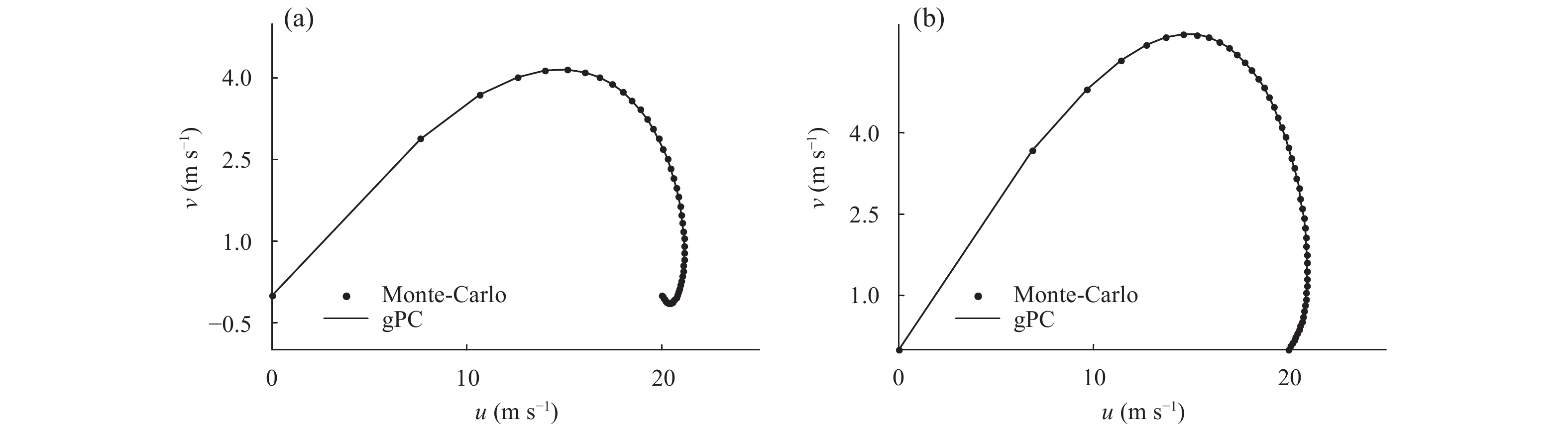Figure 15 As in Fig. 3, but for experiment Ⅲ.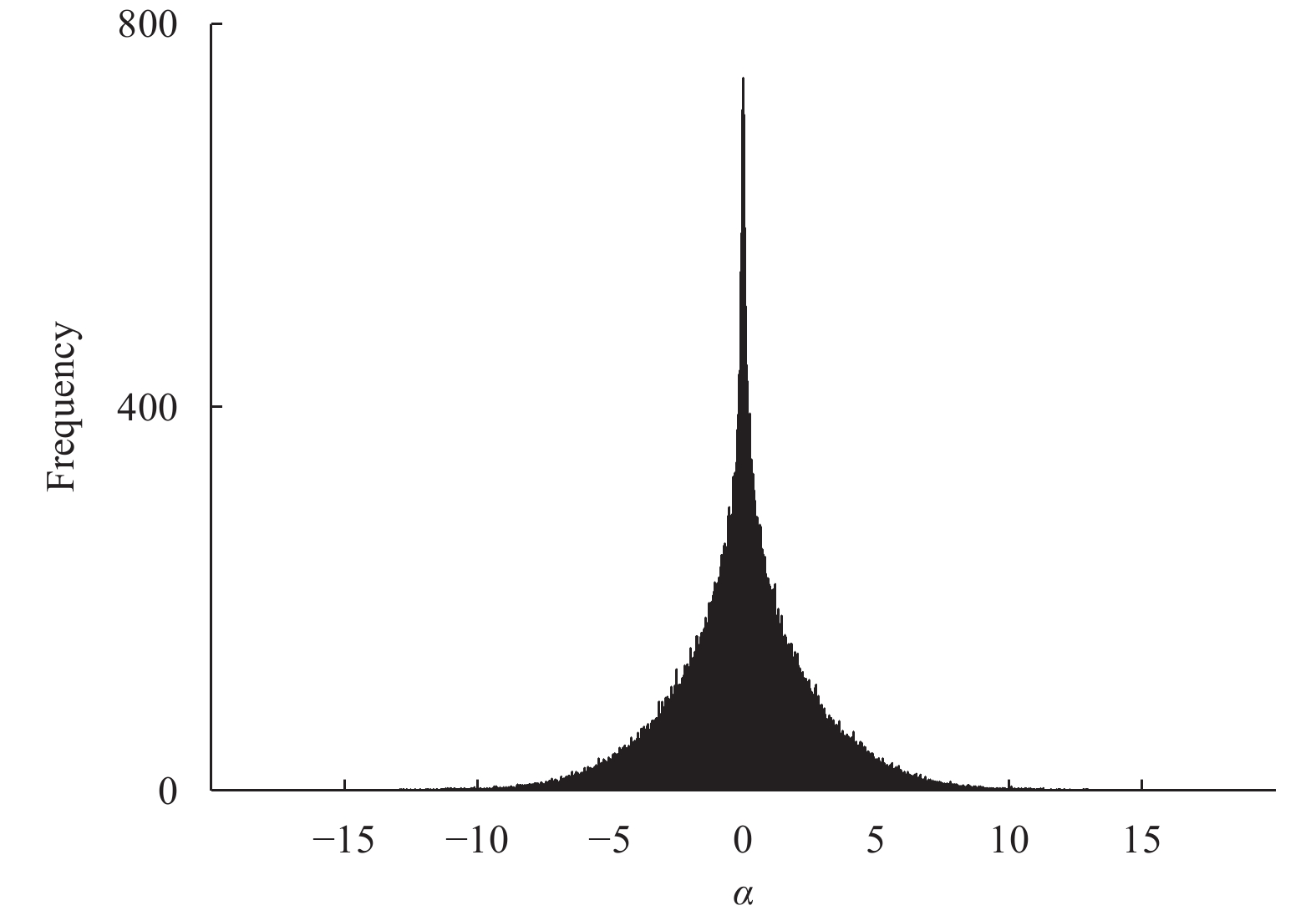Figure 14 As in Fig. 8, but for α. The abscissa is the value of α, and the ordinate is the frequency. The approximate range of α is (–10, 10), and the mean is 0.

The CPU running time and RMSE are given in Table 3. The order of the RMSE is 10–2. The CPU running time of the gPC method is far less than that of the Monte Carlo method. This finding indicates that the gPC can be used to effectively solve for the mean value of the stochastic GEM.

Table 3 The CPU time and RMSE of the two methods
 Method Cyclonic Anticyclonic CPU time RMSE CPU time RMSE gPC 0.19 0.051 0.18 0.070 Monte Carlo 6.26 0.054 6.22 0.069

Figures 16 and 17 illustrate that the differences in the standard deviations between the gPC and Monte Carlo methods are small. The gPC can effectively provide the statistical information required by the GEM with stochastic eddy viscosity. The large values of standard deviations of $u$ mainly lie at the bottom of the boundary layer, and the large values of standard deviations of $v$ appear in the central region of the boundary layer, which is less than the former.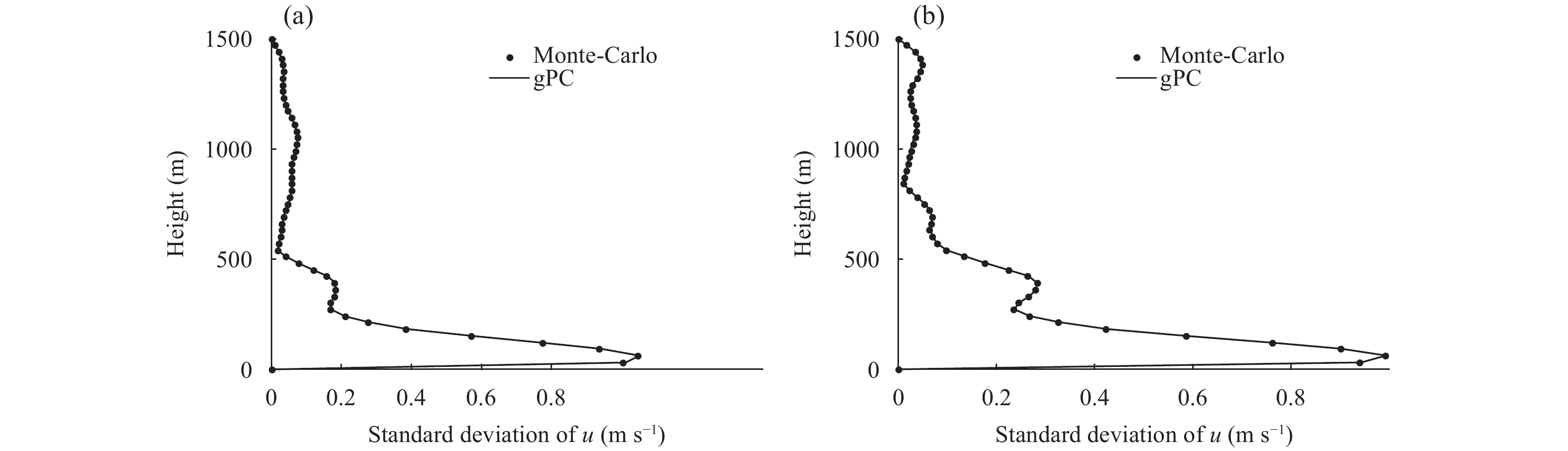Figure 16 As in Fig. 4, but for experiment Ⅲ.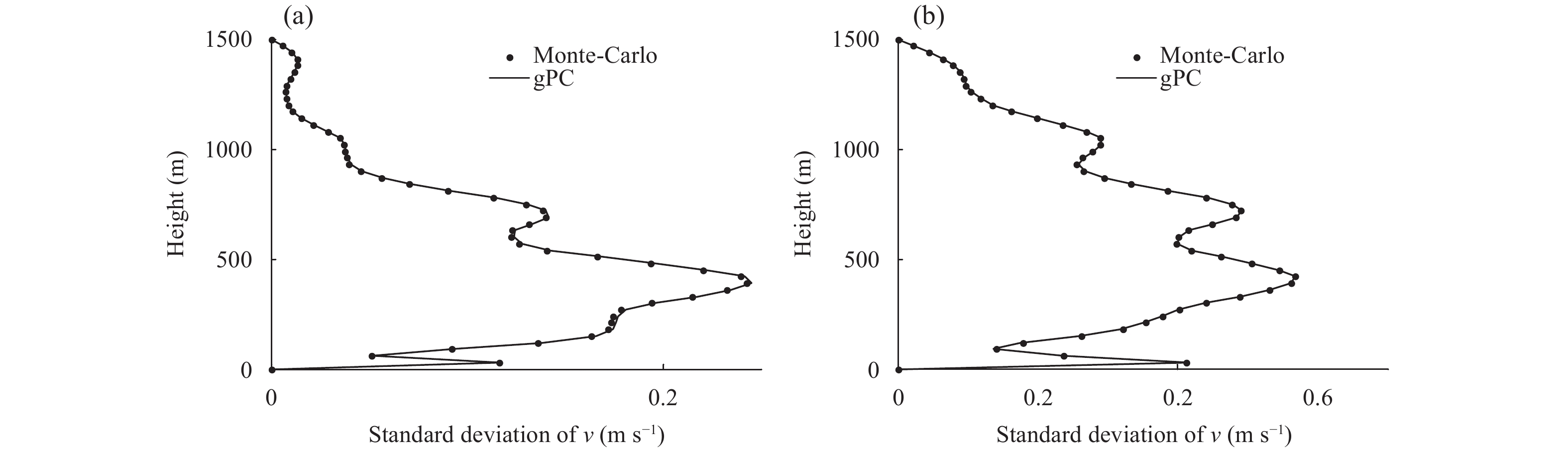Figure 17 As in Fig. 5, but for experiment Ⅲ.

It can be seen from Figs. 18 and 19 that the uncertainties of distributions are small. The large uncertainty of $u$ mainly lies at the bottom of the boundary layer, and the large uncertainty of $v$ appears in the central region of the boundary layer.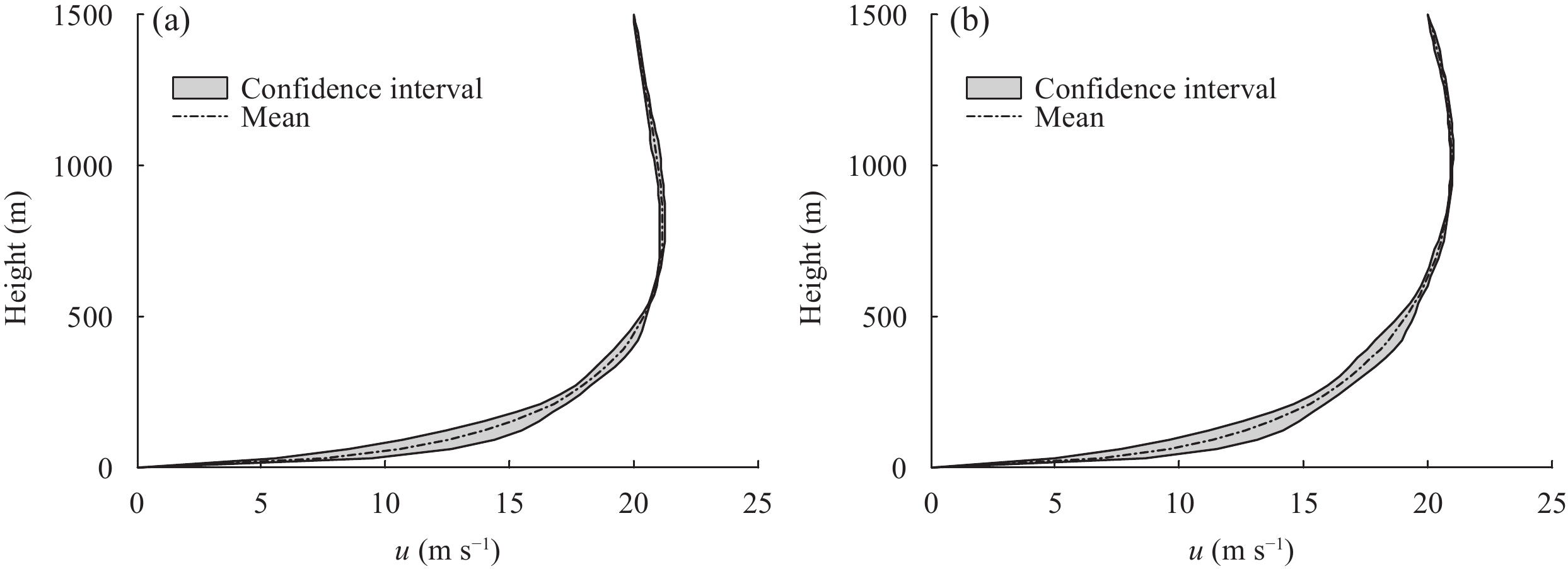Figure 18 As in Fig. 6, but for experiment Ⅲ.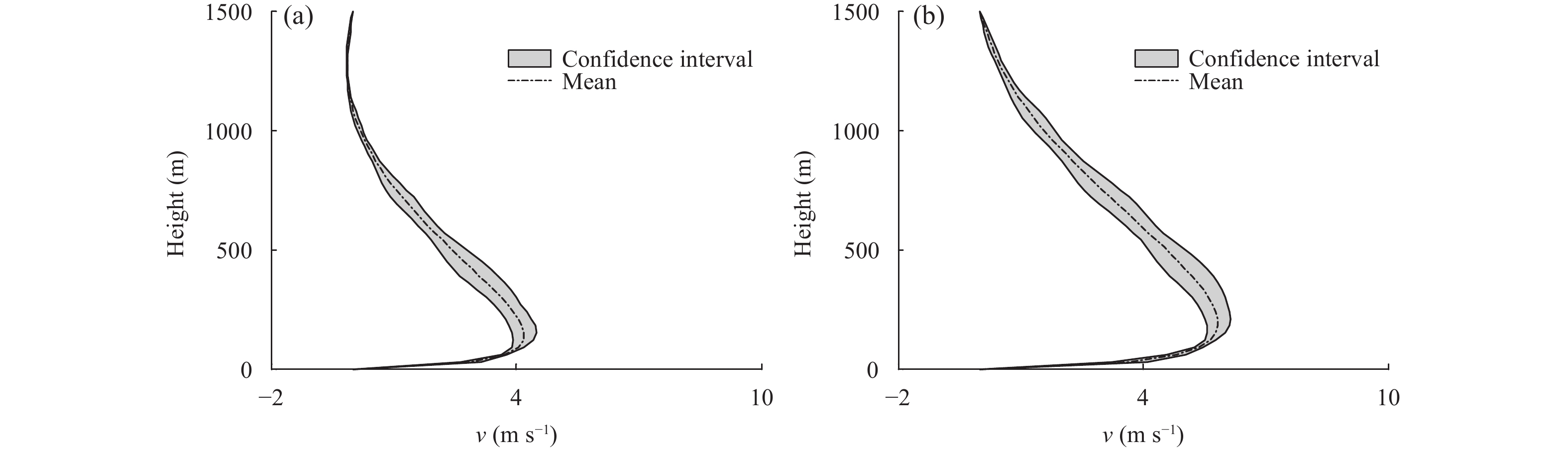Figure 19 As in Fig. 7, but for experiment Ⅲ.
5 Summary and discussion

In this paper, the eddy viscosity is replaced with a random eddy viscosity in the GEM. We quantify the uncertainty of wind in the Ekman layer through the GEM. The approaches adopted for this study are the gPC and regression method. The regression method is used to determine the expansion coefficients in the gPC expansion. Three different types of random eddy viscosities are used in the numerical tests. The results of these experiments show that the gPC combined with the regression method is a fast and accurate technique for solving the GEM with random parameter.

In this study, the random variables are assumed to be Gaussian random variables, and the range of Gaussian random variable is $(- \infty, \infty)$ . Therefore, this assumption is bound to generate $K < 0$ when the number of samples is sufficiently large, and this does not accurately reflect that of the actual atmosphere. In this paper, we avoid this by considering an appropriate standard deviation. In future studies, the truncated Gaussian distribution will be selected for research. There is a clear boundary in the truncated Gaussian distribution, and the proposed approach is more suitable for the actual situation.

Appendix

Let $(\varOmega, {\cal{F}}, P)$ be a probability space, where $\varOmega$ is a countable event space, ${\cal{F}}$ is the $\sigma \!\!\!{\text{ - }}$ field of $\varOmega$ , and $P$ is a probability measure; $\omega$ is a random event belonging to $\varOmega$ , and $\left\{ {{\xi _1}(\omega), {\xi _2}(\omega), ..., {\xi _n}(\omega)} \right\}$ are $n \!\!\!{\text{ - }}$ dimensional random variables. Additionally, $\left\{ {{\varPsi _k}({\xi _i})} \right\}$ is a system of orthogonal polynomials. Each random variable $\zeta \subset \varOmega$ is associated with a gPC expansion in the following form.

 $\begin{array}{l} \zeta (\omega) = {\zeta _0}{\varPsi _0} + \displaystyle\sum\limits_{k = 1}^\infty {{\zeta _k}{\varPsi _1}({\xi _k})} \\ + \;\displaystyle\sum\limits_{k = 1}^\infty {\displaystyle\sum\limits_{j = 1}^k {{\zeta _{kj}}{\varPsi _2}({\xi _k}, {\xi _j})} } + \;\displaystyle\sum\limits_{k = 1}^\infty {\displaystyle\sum\limits_{j = 1}^k {\displaystyle\sum\limits_{l = 1}^j {{\zeta _{kjl}}{\varPsi _3}({\xi _k}, {\xi _j}, {\xi _l})} } } + \cdots \\ \end{array} , \tag{A1}$ (A1)

where ${\xi _k} \, \, \, (k = 1, \cdots \infty)$ is mutually independent random variable, and ${\zeta _k}, {\zeta _{kj}}, {\zeta _{kjl}}, \cdots$ are gPC expansion coefficients. It is necessary to perform a finite number of computations and modify the gPC representation into a more compact form:

 $\zeta (\omega) = \sum\limits_{k = 0}^P {{\zeta _k}{\varPsi _k}( \xi)} , \tag{A2}$ (A2)

where ${\zeta _k}$ represents the deterministic gPC expansion coefficients. The number of terms retained in the expansion is as follows:

 $P + 1 = \dfrac{{(n + p)!}}{{n!p!}}, \tag{A3}$ (A3)

where $n$ is the dimension of the random variables and $p$ is the order of truncated gPC expansion. Different families of orthogonal polynomials ${\varPsi _k}( \xi)$ can be chosen to achieve better convergence for different types of probability distribution, and they are determined by using the hypergeometric series and incorporated in the Askey scheme (Schoutens, 2000). Part of the probability distribution and the corresponding orthogonal polynomials are shown in Table A1.

Table A1 Probability distribution and the corresponding polynomials
 Distribution ( $\xi$ ) Polynomial ( $\varPsi$ ) Support Continuous Gamma Laguerre $\left[ {0, \infty } \right)$ Beta Jacobi $\left[ {a, b} \right]$ Uniform Legendre $\left[ {a, b} \right]$ Gaussian Hermite $\left({ - \infty, \infty } \right)$ Discrete Negative binomial Meixner $\left\ (0, 1, 2, \cdots ) \right\$ Binomial Krawtchouk $\left( {0, 1, 2, \cdots, N} \right)$ Poisson Charlier $\left( {0, 1, 2, \cdots } \right)$ Hypergeometric Hahn $\left( {0, 1, 2, \cdots, N} \right)$

The following is an example of Hermite polynomial chaos corresponding to normal distribution. It is well known that orthogonal polynomials satisfy a three-term recurrence relation

 ${\varPsi _{n + 1}}(\xi) = \xi \, {\varPsi _n}(\xi) - n{\varPsi _{n - 1}}(\xi), \quad n > 0, \tag{A4}$ (A4)

where ${\varPsi _0} = 1$ and ${\varPsi _1} = \xi$ . Thus, we can get the specific forms of ${\varPsi _n}(\xi), \;n > 0$ . After that, the multivariate polynomial chaos can be obtained by graded lexicographic order method (Xiu, 2010).

References
 Baklanov, A. A., B. Grisogono, R. Bornstein, et al., 2011: The nature, theory, and modeling of atmospheric planetary boundary layers. Bull. Amer. Meteor. Soc., 92, 123–128. DOI:10.1175/2010BAMS2797.1 Barzel, B., and O. Biham, 2011: Binomial moment equations for stochastic reaction systems. Phys. Rev. Lett., 106, 150602. DOI:10.1103/PhysRevLett.106.150602 Beare, R. J., and M. J. P. Cullen, 2010: A semi-geostrophic mo-del incorporating well-mixed boundary layers. Quart. J. Roy. Meteor. Soc., 136, 906–917. DOI:10.1002/qj.612 Berger, B. W., and B. Grisogono, 1998: The baroclinic, variable eddy viscosity Ekman layer. Bound.-Layer Meteor., 87, 363–380. DOI:10.1023/A:1001076030166 Berner, J., U. Achatz, L. Batté, et al., 2017: Stochastic parameterization: Toward a new view of weather and climate models. Bull. Amer. Meteor. Soc., 98, 565–588. DOI:10.1175/bams-d-15-00268.1 Blumen, W., and R. S. Wu, 1983: Baroclinic instability and frontogenesis with Ekman boundary layer dynamics incorporating the geostrophic momentum approximation. J. Atmos. Sci., 40, 2630–2638. DOI:10.1175/1520-0469(1983)040<2630:BIAFWE>2.0.CO;2 Cullen, M. J. P., 1989: On the incorporation of atmospheric boundary layer effects into a balanced model. Quart. J. Roy. Meteor. Soc., 115, 1109–1131. DOI:10.1002/qj.49711548906 D’Onofrio, L., A. Fiscella, and G. M. Bisci, 2017: Perturbation methods for nonlocal Kirchhoff-type problems. Fract. Calc. Appl. Anal., 20, 829–853. DOI:10.1515/fca-2017-0044 Ekman, V. W., 1905: On the influence of the earth’s rotation on ocean-currents. Arch. Math. Astron. Phys., 2, 1–53. Fox, B. L., 1999: Strategies for Quasi-Monte Carlo. Springer, Boston, 54–93, doi: 10.1007/978-1-4615-5221-5. Ghanem, R. G., and P. D. Spanos, 1991: Stochastic Finite Elements: A Spectral Approach. Springer, New York, 46–105, doi: 10.1007/978-1-4612-3094-6. Grisogono, B., T. Jurlina, Ž. Večenaj, et al., 2015: Weakly nonlinear Prandtl model for simple slope flows. Quart. J. Roy. Meteor. Soc., 141, 883–892. DOI:10.1002/qj.2406 Helton, J. C., and F. J. Davis, 2003: Latin hypercube sampling and the propagation of uncertainty in analyses of complex systems. Reliability Engineering & System Safety, 81, 23–69. DOI:10.1016/S0951-8320(03)00058-9 Le Maître, O. P., and O. M. Knio, 2010: Spectral Methods for Uncertainty Quantification: With Applications to Computational Fluid Dynamics. Springer, Dordrecht, 18–29, doi: 10.1007/978-90-481-3520-2. Li, J., and D. B. Xiu, 2009: A generalized polynomial chaos based ensemble Kalman filter with high accuracy. J. Comput. Phys., 228, 5454–5469. DOI:10.1016/j.jcp.2009.04.029 Li, W. X., Z. M. Lu, and D. X. Zhang, 2009: Stochastic analysis of unsaturated flow with probabilistic collocation method. Water Resour. Res., 45, W08425. DOI:10.1029/2008WR007530 Li, W. X., G. Lin, and D. X. Zhang, 2014: An adaptive ANOVA-based PCKF for high-dimensional nonlinear inverse modeling. J. Comput. Phys., 258, 752–772. DOI:10.1016/j.jcp.2013.11.019 Lin, G., A. M. Tartakovsky, and D. M. Tartakovsky, 2010: Uncertainty quantification via random domain decomposition and probabilistic collocation on sparse grids. J. Comput. Phys., 229, 6995–7012. DOI:10.1016/j.jcp.2010.05.036 Loh, W. L., 1996: On Latin hypercube sampling. Ann. Stat., 24, 2058–2080. DOI:10.1214/aos/1069362310 Mahrt, L., 1998: Stratified atmospheric boundary layers and breakdown of models. Theoret. Comput. Fluid Dynamics, 11, 263–279. DOI:10.1007/s001620050093 Marlatt, S., S. Waggy, and S. Biringen, 2012: Direct numerical simulation of the turbulent Ekman layer: Evaluation of closure models. J. Atmos. Sci., 69, 1106–1117. DOI:10.1175/JAS-D-11-0107.1 Marzouk, Y. M., and H. N. Najm, 2009: Dimensionality reduction and polynomial chaos acceleration of Bayesian inference in inverse problems. J. Comput. Phys., 228, 1862–1902. DOI:10.1016/j.jcp.2008.11.024 Najm, H. N., 2009: Uncertainty quantification and polynomial chaos techniques in computational fluid dynamics. Annu. Rev. Fluid Mech., 41, 35–52. DOI:10.1146/annurev.fluid.010908.165248 Schoutens, W., 2000: The Askey scheme of orthogonal polynomials. Stochastic Processes and Orthogonal Polynomials, W. Schoutens, Ed., Springer, New York, 1–13, doi: 10.1007/978-1-4612-1170-9. Singer, H., 2006: Moment equations and Hermite expansion for nonlinear stochastic differential equations with application to stock price models. Comput. Stat., 21, 385–397. DOI:10.1007/s00180-006-0001-4 Sun, N. Z., and A. Sun, 2015: Model uncertainty quantification. Model Calibration and Parameter Estimation: For Environmental and Water Resource Systems, N. Z. Sun, and A. Sun, Ed., Springer, New York, 407–458, doi: 10.1007/978-1-4939-2323-6_10. Tan, Z. M., 2001: An approximate analytical solution for the baroclinic and variable eddy diffusivity semi-geostrophic Ekman boundary layer. Bound.-Layer Meteor., 98, 361–385. DOI:10.1023/A:1018708726112 Tan, Z. M., and R. S. Wu, 1994: The Ekman momentum approximation and its application. Bound.-Layer Meteor., 68, 193–199. DOI:10.1007/BF00712671 Tan, Z. M., and Y. Wang, 2002: Wind structure in an intermediate boundary layer model based on Ekman momentum approximation. Adv. Atmos. Sci., 19, 266–278. DOI:10.1007/s00376-002-0021-0 Tan, Z. M., J. Fang, and R. S. Wu, 2006: Nonlinear Ekman layer theories and their applications. J. Meteor. Res., 20, 209–222. Twigg, R. D., and P. R. Bannon, 1998: Frontal equilibration by frictional processes. J. Atmos. Sci., 55, 1084–1087. DOI:10.1175/1520-0469(1998)055<1084:FEBFP>2.0.CO;2 Wang, Y. P., Y. Cheng, Z. Y. Zhang, et al., 2018: Calibration of reduced-order model for a coupled Burgers equations based on PC-EnKF. Math. Model. Nat, Phenom., 13, 21. DOI:10.1051/mmnp/2018023 Wiener, N., 1938: The homogeneous chaos. Amer. J. Math., 60, 897–936. DOI:10.2307/2371268 Wu, R. S., and W. Blumen, 1982: An analysis of Ekman boundary layer dynamics incorporating the geostrophic momentum approximation. J. Atmos. Sci., 39, 1774–1782. DOI:10.1175/1520-0469(1982)039<1774:AAOEBL>2.0.CO;2 Xiu, D. B., 2009: Fast numerical methods for stochastic computations: A review. Comput. Commun. Phys., 5, 242–272. Xiu, D. B., 2010: Numerical Methods for Stochastic Computations: A Spectral Method Approach. Princeton University Press, Princeton, 26–88. Yan, B., S. X. Huang, and J. Feng, 2017: Retrieval of eddy thermal conductivity in the weakly nonlinear Prandtl model for katabatic flows. J. Meteor. Res., 31, 965–975. DOI:10.1007/s13351-017-7025-2 Yan, B., S. X. Huang, and J. Feng, 2018: Retrieval and uncertainty analysis of stochastic parameter in atmospheric boundary layer model. Acta Phys. Sinica, 67, 199201. DOI:10.7498/aps.67.20181014 Zeng, L. Z., and D. X. Zhang, 2010: A stochastic collocation based Kalman filter for data assimilation. Comput. Geosci., 14, 721–744. DOI:10.1007/s10596-010-9183-5